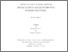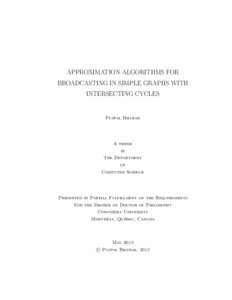Title:

# Approximation Algorithms for Broadcasting in Simple Graphs with Intersecting Cycles

Bhabak, Puspal (2014) Approximation Algorithms for Broadcasting in Simple Graphs with Intersecting Cycles. PhD thesis, Concordia University.Preview Text (application/pdf) Thesis_PuspalBhabak.pdf - Accepted Version 1MB

## Abstract

Broadcasting is an information dissemination problem in a connected network in which one node, called the originator, must distribute a message to all other nodes
by placing a series of calls along the communication lines of the network. Every time the informed nodes aid the originator in distributing the message. Finding the
minimum broadcast time of any vertex in an arbitrary graph is NP-Complete. The problem remains NP-Complete even for planar graphs of degree 3 and for a graph
whose vertex set can be partitioned into a clique and an independent set. The best theoretical upper bound gives logarithmic approximation. It has been shown that
the broadcasting problem is NP-Hard to approximate within a factor of 3-ɛ. The polynomial time solvability is shown only for tree-like graphs; trees, unicyclic graphs,
tree of cycles, necklace graphs and some graphs where the underlying graph is a clique; such as fully connected trees and tree of cliques. In this thesis we study the
broadcast problem in different classes of graphs where cycles intersect in at least one vertex. First we consider broadcasting in a simple graph where several cycles have common paths and two intersecting vertices, called a k-path graph. We present a constant approximation algorithm to find the broadcast time of an arbitrary k-path graph. We also study the broadcast problem in a simple cactus graph called k-cycle
graph where several cycles of arbitrary lengths are connected by a central vertex on one end. We design a constant approximation algorithm to find the broadcast time of an arbitrary k-cycle graph.
Next we study the broadcast problem in a hypercube of trees for which we present a 2-approximation algorithm for any originator. We provide a linear algorithm to
find the broadcast time in hypercube of trees with one tree. We extend the result for any arbitrary graph whose nodes contain trees and design a linear time constant approximation algorithm where the broadcast scheme in the arbitrary graph is already known.
In Chapter 6 we study broadcasting in Harary graph for which we present an additive approximation which gives 2-approximation in the worst case to find the broadcast time in an arbitrary Harary graph. Next for even values of n, we introduce a new graph, called modified-Harary graph and present a 1-additive approximation
algorithm to find the broadcast time. We also show that a modified-Harary graph is a broadcast graph when k is logarithmic of n.
Finally we consider a diameter broadcast problem where we obtain a lower bound on the broadcast time of the graph which has at least (d+k-1 choose d) + 1 vertices that are at a distance d from the originator, where k >= 1.

Divisions: Concordia University > Gina Cody School of Engineering and Computer Science > Computer Science and Software Engineering Thesis (PhD) Bhabak, Puspal Concordia University Ph. D. Computer Science 12 December 2014 Harutyunyan, Hovhannes Approximation Algorithm Broadcasting Networks NP-Hard 979568 PUSPAL BHABAK 16 Jul 2015 12:50 18 Jul 2019 15:06
All items in Spectrum are protected by copyright, with all rights reserved. The use of items is governed by Spectrum's terms of access.

Repository Staff Only: item control page

Research related to the current document (at the CORE website)Research related to the current document (at the CORE website)
Back to top